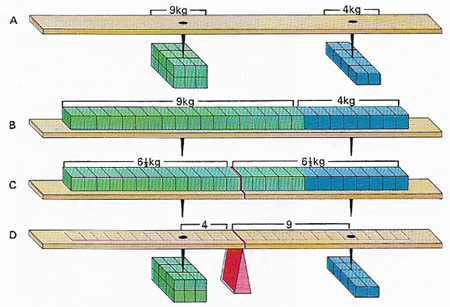A# forceFig 1. The balance point of a lever (itself assumed weightless) (A) can be found by dividing each load into equal small weights and distributing them along the lever (B), keeping the center of gravity of each load where it was. The lever balances at the center of gravity of the whole line. This point is halfway along the line (C). of equal weights at the distance from the weights that is inverse to the ratio of the weights themselves (D). The product of the weight and its distance from the fulcrum (pivot) is the same on each side – the moments are equal and opposite.

A force is the cause of a change in motion. It may start an object moving when it's been at rest, or stop an object that's been moving. A force may also change a condition of motion that already exists, resulting in an acceleration, deceleration, or change of direction. Once made to move, an object continues to move without stopping or changing direction until it is acted upon by another force. The idea has now become almost self-evident following its original expression and generalization by Isaac Newton in the late 1600s.

Force is defined by Newton's second law as the rate of change of momentum or, equivalently, as the product of mass and acceleration (F = m × a). It is a vector quantity, in the direction of the acceleration it brings about. Forces always occur in equal and opposite action-reaction pairs between bodies, though it is often useful to think of one body being in a force field.

The SI (international system) unit of force is the newton (N). For example, if a 5-kg block is sitting at rest and is then pushed horizontally with an initial acceleration of 3 m/s2, the force that sets the block in motion is 15 N (5 kg × 3 m/s2).

## The importance of friction

In many situations on Earth, the cause that stops, or modifies, already existing motion is provided by the force of friction. This acts in the direction opposite to the movement of an object. It is produced by the rubbing together of the surface of the moving object and the surface it is moving on or, in a gas or liquid, the medium it is moving through. We're so used to seeing how objects behave when friction is present that their behavior in space, or in other airless environments, seems strange.

## States of equilibrium

When several forces act at the same time on the same object, each tries to move the object along a line pointing in its own direction at a rate that depends on the size of the applied force. If it happens that the object does not move as a result of all these forces, then it is said to be in equilibrium (Fig 1).

The magnitude and direction of any of any one of the forces is balanced by the total effect of the other forces and there is no resultant movement. The study of forces applied to objects in a state of equilibrium is called statics, as opposed to dynamics, the study of forces acting on moving objects.

Someone sitting still on a chair is an everyday example of an object in equilibrium – the upward force of the chair on the person balances the downward force of Earth's gravitational attraction that is trying to pull the person through the chair to the floor. A tree standing upright in the ground is a similar example – its downward gravitational force, commonly known as its weight, is balanced by the upward force of the ground in which it is rooted. As a consequence of this equilibrium, neither the person nor the tree is in motion up or down. This state of affairs can be modified only if another external force is brought into the arrangement – for example, by the person moving moving about on the chair and possibly making it topple or, in a most extreme case, by chopping down the tree.

## Moments and levers

So far, only those forces that try to move objects along straight-line paths have been considered. There are many other forces, however, that can act on objects and try to rotate them around a central point. These forces have an effectiveness that depends on how far from the central point they are acting. See moment and lever.